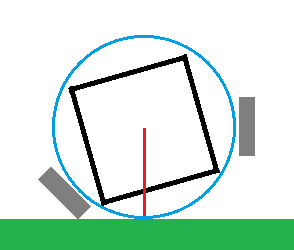# How to determine the center of gravity of a satellite

• I
• Romka
The CG of a CubeSat is located at the bottom of the sphere when it is on a flat surface. To measure the CG, the CubeSat is hit with a golf putter. Then it is put on a slippery surface and hit with a 9 iron. Finally, it is put on a golf tee and hit with a 9 iron.

#### Romka

Hello,

I am trying to find out how to determine the center of gravity of a CubeSat present in an uniform sphere of radius R, using the initial data given by gyroscopes. The satelitte is equipped with magnetometers, gyroscopes, and accelerometers. By taking into account that the only values I have are angular rates around the three axes, it seems that both the Newton's laws and equations of motion are not enough.
Does anybody have any idea?

I'm going to assume that the sphere is massless. Otherwise, we'll be determining the CG (center of gravity) of the combined sphere and satellite.

Step one would be to allow the sphere to role to a rest on a flat horizontal surface. This would put the CG at it lowest point - directly over the point where the sphere touches the supporting surface. The accelerometers would then tell you how the cube was tilted, thus providing a line where the CG must fall.

That "low point" on the sphere will also be used as the bottom of the sphere in the next two steps.

Step two would be to put it on a slippery (frictionless) horizontal surface and hit it with a golf putter - directing a horizontal force on it. The low CG will cause it to spin - and the instrumentation will be able to measure the acceleration and angular velocity.

Finally, put it onto a golf tee and hit it with a frictionless 9 iron (a 45-degree right ascension angle). Again, the instrumentation will be able to measure the acceleration and angular velocity.CG will be along the red line segment.
The gray blocks are the frictionless golf heads. One a putter, the other a 9-iron (45 degrees).

Unless the CG is exactly in the middle of the sphere, each golf strike will cause a top spin proportional to the increased velocity.

Now that I look at it, only one golf strike should be needed to compute the CG. Calculations should be simpler and more accurate with the putter.

#### Attachments

Last edited:
Why not measure the center of gravity before launch?
But nevertheless, I suppose this is a situation where the inverse problem is easier to solve. Given a constant velocity of the center of mass and constant angular momentum, and given the location of the sensors relative to the center of mass, it should be easy to calculate the accelerations and rotations of the sensors as a function of time. You will need to know the location of the sensors relative to each other, at least. Then you can numerically solve for the best fit location of the center of mass based on your data.

## 1. How is the center of gravity of a satellite determined?

The center of gravity of a satellite is determined by finding the average location of all the mass in the satellite. This can be done by calculating the mass of each component of the satellite and its distance from a fixed reference point, then using those values to calculate the overall center of gravity.

## 2. What factors affect the center of gravity of a satellite?

The main factors that affect the center of gravity of a satellite are the distribution and arrangement of its mass. The location and mass of each component, as well as any external forces acting on the satellite, can also impact its center of gravity.

## 3. Why is it important to determine the center of gravity of a satellite?

Determining the center of gravity of a satellite is crucial for ensuring its stability and proper functioning in orbit. A satellite with an incorrect center of gravity may experience issues such as tumbling or loss of control, which can affect its ability to perform its intended tasks.

## 4. How is the center of gravity of a satellite different from its center of mass?

The center of gravity and center of mass of a satellite are often used interchangeably, but they are not exactly the same. The center of gravity refers to the average location of the mass in a satellite, while the center of mass refers to the point where the entire mass of the satellite can be considered to be concentrated.

## 5. Can the center of gravity of a satellite change over time?

Yes, the center of gravity of a satellite can change over time due to various factors such as changes in the distribution of mass or external forces acting on the satellite. It is important to regularly monitor and adjust the center of gravity of a satellite to ensure its stability and proper functioning in orbit.